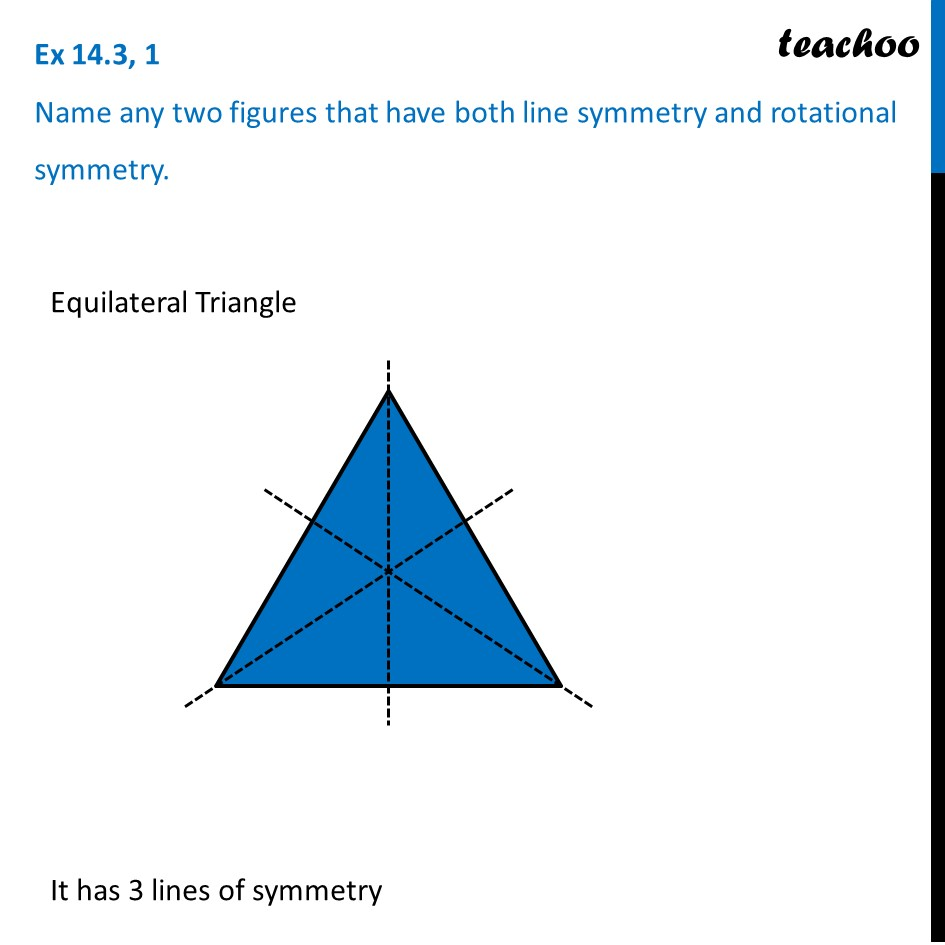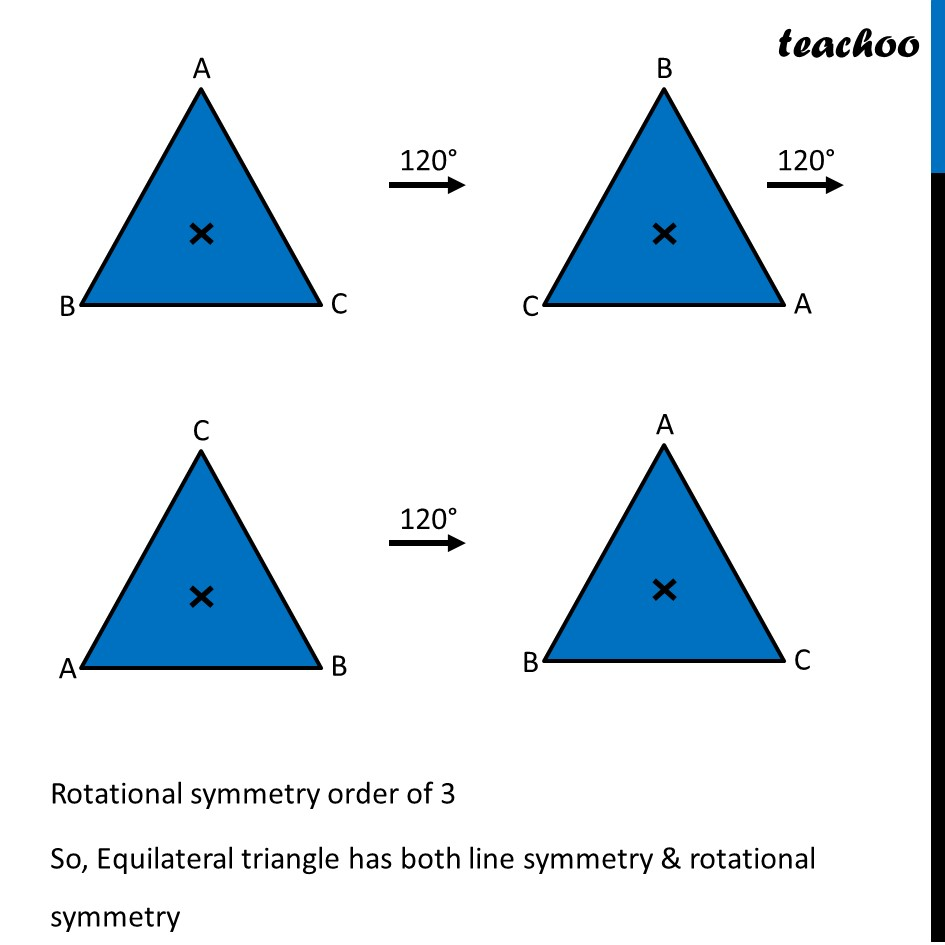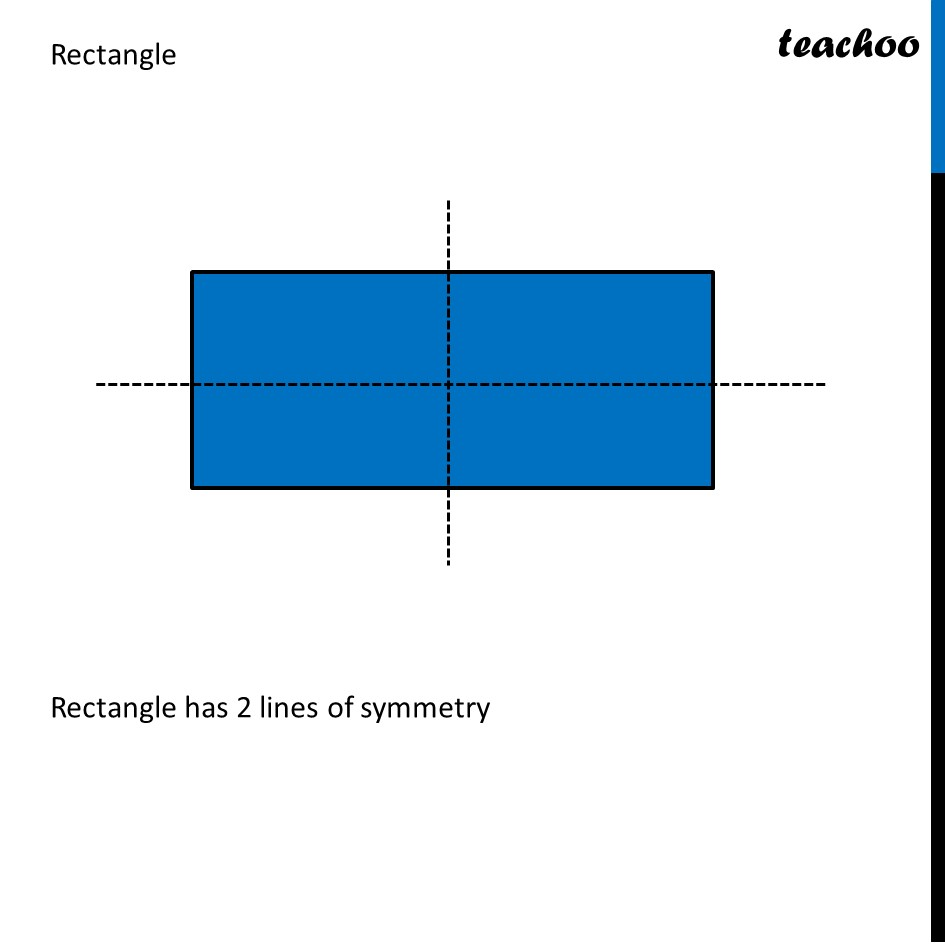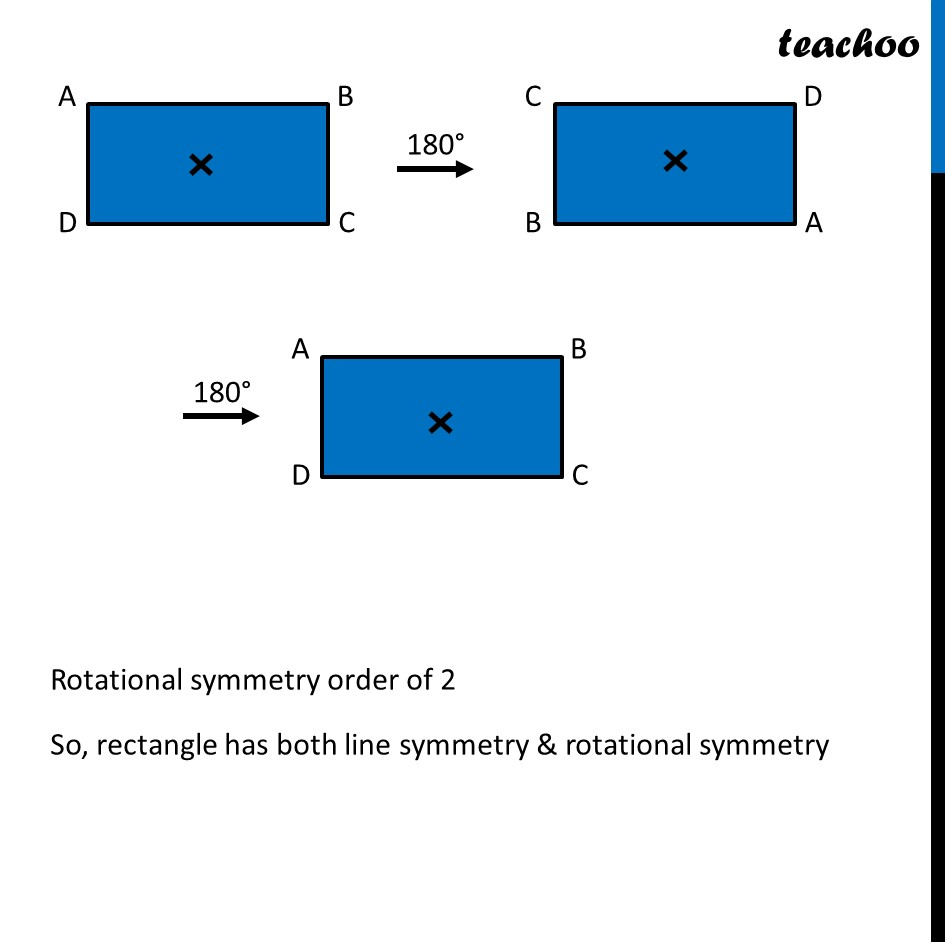Ex 14.3

Chapter 14 Class 7 Symmetry
Serial order wise### Transcript

Ex 14.3, 1 Name any two figures that have both line symmetry and rotational symmetry.Equilateral Triangle It has 3 lines of symmetry Rotational symmetry order of 3 So, Equilateral triangle has both line symmetry & rotational symmetry Rectangle Rectangle has 2 lines of symmetry Rotational symmetry order of 2 So, rectangle has both line symmetry & rotational symmetry